• 如何在PYNQ和ZYNQ上用FPGA加速神经网络
千次阅读
2020-07-30 16:46:46

转载https://blog.csdn.net/qq_35999634/article/details/81672331

如何在PYNQ和ZYNQ上用FPGA加速神经网络

2. 目前有两个例程，都是ARM+FPGA：a、Spark+PYNQ+LR进行MNIST模型训练；b、Theano+CNN进行MNIST模型预测。且都对比了有FPGA加速和仅用ARM时的速度。
3. ZYNQ加速和PYNQ加速大体基本一致，就是在顶层用C/C++调用已在ZYNQ上配置好的新框架和IP

## 公众号：https://mp.weixin.qq.com/s/mipdI1iVJiaIAtJTGA4oWQ

更多相关内容
• 优秀的 Verilog/FPGA开源项目介绍（十五）- 加速神经网络的必备开源项目之前介绍的项目《优秀的 Verilog/FPGA开源项目介绍（十四）- 使用FPGA实现LeNet-5 深...

# 优秀的 Verilog/FPGA开源项目介绍（十五）- 加速神经网络的必备开源项目之前介绍的项目《优秀的 Verilog/FPGA开源项目介绍（十四）- 使用FPGA实现LeNet-5 深度神经网络模型》最后我们分析了，纯FPGA实现神经网络的缺点，以及现在FPGA厂家的加速方案，这里引用一下：

到底纯FPGA适不适合这种大型神经网络的设计？这个问题其实我们不适合回答，但是FPGA厂商是的实际操作是很有权威性的，现在不论是Intel还是Xilinx都没有在自己传统的FPGA上推广AI，都是在基于FPGA的SoC上推广（Vitis和OpenVINO，前者Xilinx后者Intel），总结来看就是：纯 RTL 硬件设计不是AI的好选择。特别是对于大规模网络，权重和中间结果需要存储在外部存储器中。并且数据迭代器会更加复杂。设计周期长，AI相关领域迭代速度快，综上以上几点，可以很容易给你们指引一条道路。

目前主流的解决方案就是使用通用或专用处理器来做控制工作，让硬件来执行计算（加速的概念），今天就介绍两个针对以上解决方案的开源项目，这两个项目是用FPGA进行硬件加速的必备项目。

# AI算法流程

在进行项目介绍前，我们先介绍一下软件架构和工具集。这个后面会影响理解。

一个完整的深度学习框架中主要分为下面几个流程：

展开全文算法 大数据 编程语言
• 基于FPGA神经网络CNN加速器，基于FPGA神经网络CNN加速器，基于FPGA神经网络CNN加速器，基于FPGA神经网络CNN加速器，基于FPGA神经网络CNN加速器，基于FPGA神经网络CNN加速器。
• FPGA加速卷积神经网络的知识储备1.FPGA1.FPGA分类2.开发工具3.开发工具22.卷积神经网络1.卷积神经网络基础2.开发语言3.总结参考内容 本文是我经过一段时间学习的学习总结，由于是初学者的缘故，可能总结会有纰漏...

### 用FPGA加速卷积神经网络的知识储备

本文是我经过一段时间学习的学习总结，由于是初学者的缘故，可能总结会有纰漏甚至错误，希望同样从事该方向研究的大佬以及爱好者批评指正，不胜感激。

下面我会从硬件和神经网络两方面来介绍使用FPGA做卷积神经网络加速所需要的知识基础。

# 1.FPGA

## 1.FPGA分类

毫无疑问，Xilinx是当前FPGA领域的带头大哥，占据了百分之60左右的全球市场份额。而Altera在被intel收购以后，也有着相当优秀的发展潜力。就这两家而言，Xilinx胜在高端性能，资源丰富。而Altera胜在价格低廉，是新手入门的性价比的不二之选。

## 2.开发工具

Altera：Quartus II，集成了modelsim工能，非常直觉化，容易入门，bug很少（曾是高管骄傲的谈资），非常适合新手入门，但并不适合大规模系统开发。

## 3.开发工具2

VHDL/Verilog：硬件描述语言，永远的神。就目前而言，想要完全发挥FPGA的性能，硬件描述语言无疑是首选，但是上手有一定的难度，需要付出比较大的努力。
（补充：学习资源丰富，不会增加搜寻资源的时间成本）。

HLS：HLS是高层综合（High level Synthesis），是将C或者c++语言编译为FPGA能够读懂和运行的RTL级别的语言。通过HLS这个过程可以显著加快FPGA的设计进程，而不用从底层的FPGA语言编起。但是由于软件工程师本身的知识体系的原因，使用hls相比硬件工程师而言，仍有不小的差距。换言之，现在使用hls炉火纯青的工程师仍是硬件工程师。

OpenCL：OpenCL（全称Open CompuTIng Language，开放运算语言）是第一个面向异构系统通用目的并行编程的开放式、免费标准，也是一个统一的编程环境，便于软件开发人员为高性能计算服务器、桌面计算系统、手持设备编写高效轻便的代码，而且广泛适用于多核心处理器（CPU）、图形处理器（GPU）、Cell类型架构以及数字信号处理器（DSP）等其他并行处理器，在游戏、娱乐、科研、医疗等各种领域都有广阔的发展前景。
基于FPGA的OpenCL有如下优点：

a.与传统的FPGA设计流程相比，产品能够更迅速面市。
b.使用OpenCL C （基于ANSI C）并行编程语言而不是传统的底层硬件描述语言（HDL）来描述您的算法。
c.在更高层的设计抽象环境中迅速进行设计开发。
d.针对目前和未来的FPGA重新定位OpenCL C代码，设计不会过时。
e.跳过耗时的手动时序收敛以及FPGA、主机和外部存储器之间的通信接口设计工作，一个步骤中就可以在FPGA上实现OpenCL的C代码。
f.相比于硬件方案，功耗有明显的降低。


当然，OpenCL的缺点同HLS类似，相比于硬件描述语言，仍无法完全发挥FPGA的性能。
（补充：选择OpenCL必然选择Altera开发器件，但是目前能找到的开源资源很少，需要自身有一定的资源支撑）。

# 2.卷积神经网络

由于FPGA开发硬件的特殊性，普通的卷积神经网络直接移植到开发板上明显是达不到加速的效果的，所以想要实现加速效果，我们必须对卷积神经网络进行轻量化设计。而轻量化网络，需要我们对神经网络结构有深入了解以及对编写语言有一定的基础。

## 1.卷积神经网络基础

轻量化卷积神经网络有以下方法：如网络剪枝、低秩分解、压缩编码等，减小神经网络模型参数量、简化卷积神经网络算法运算过程等。进行以上操作，需要我们对神经网络的结构有很深入的了解，所以，实现神经网络的FPGA加速器设计，对神经网络模型的把控必不可少。
推荐学习内容：吴恩达的深度学习，李沐的动手学深度学习等。

## 2.开发语言

可以编写神经网络开发语言很多，C，C++，Python，选择哪一种开发语言需要根据上面开发工具选择相匹配，如果使用HLS或者是OpenCL，C和C++是最好的选择，如果选择硬件描述语言来设计硬件的话，那么选择Python毫无疑问更方便，因为Python相比其他两种更容易编写，更容易理解。

# 3.总结

用FPGA做神经网络的加速器，我们可以选择的方案方法搭配有很多，我们应该根据自身的能力以及所拥有的资源选择最适合自己的结合，避免时间成本的增加。在查漏补缺的同时，代码能力必然需要提高！代码能力必然需要提高！！代码能力必然需要提高！！！（重要的事情说三遍）代码是灵魂，没有代码能力，一切都是空谈！！！！！！

最后，还是希望同方向的大佬以及爱好者批评指正，分享资源，不胜感激。

# 参考内容

参考内容：http://www.elecfans.com/emb/fpga/20130412313552.html

展开全文• bnn-fpgaFPGA上CIFAR-10的二进制神经网络（BNN）加速器的开源实现。 加速器针对低功耗嵌入式现场可编程SoC，并在Zedboard上进行了测试。 在编写CIFAR-10测试集中的10000张图像时，错误率是11.19％。
•研究论文
• 可以看到，该模块由三个卷积组成，第一第三个卷积是标准的1x1卷积，起到升维和降维的作用，而中间的是一个depthwise卷积，每一个卷积层之后，都紧接着一个BN层，以加速网络的收敛。 同时，我们观察到，该模块的输入...

# MobileNet V2介绍

MobileNetV2是在V1基础之上的改进。V1主要思想就是深度可分离卷积。而V2则在V1的基础上，引入了Linear BottleneckInverted Residuals。下图是MobileNet V2中的一个基本模块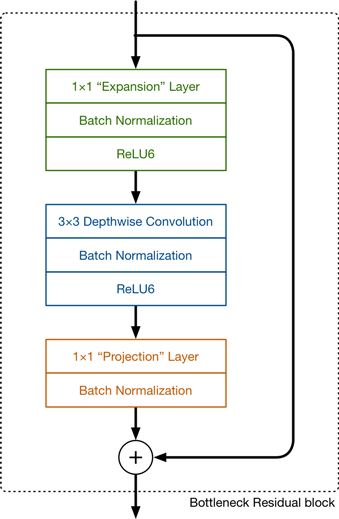可以看到，该模块由三个卷积组成，第一第三个卷积是标准的1x1卷积，起到升维和降维的作用，而中间的是一个depthwise卷积，每一个卷积层之后，都紧接着一个BN层，以加速网络的收敛。
同时，我们观察到，该模块的输入和输出有一个残差连接，即输入和最终的输出求和，这就是MobileNet V2中的Inverted Residuals，同时，我们注意到，第三个卷积层之后，并没有跟着一个激活函数，这就是上面所说的Linear Bottleneck，因为从高维向低维转换时，使用ReLU激活函数可能会造成信息丢失或破坏（不使用非线性激活数数）。所以在最后一个卷积之后，我们不再使用ReLU激活函数而是使用线性激活函数。
有了上边的基础，整体的网络结构就很好理解了，如下图所示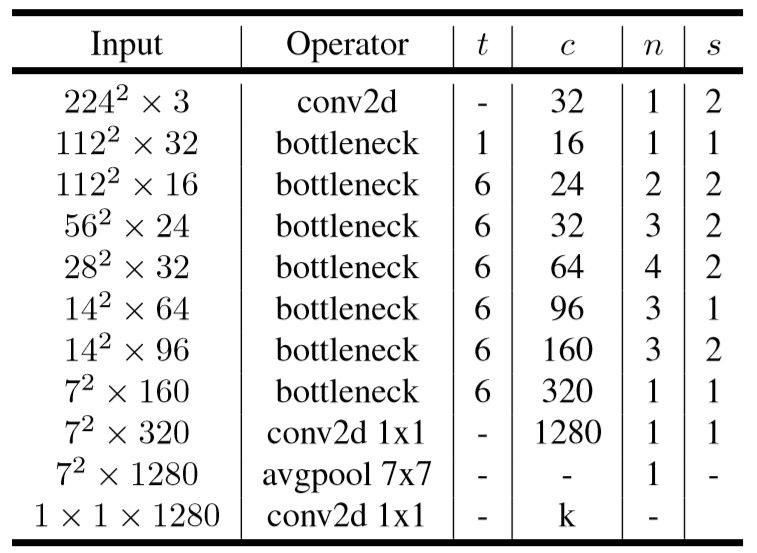下图则是MobileNet V2和其他网络在参数量、计算复杂度方面的对比，可以看到，MobileNet V2的参数数目为3.4M，乘累加数目为300M.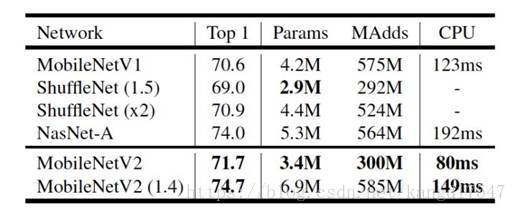# 模型训练以及参数预处理

模型训练，我们采用的是一个有5种花卉类别的数据集，输入图像的尺寸为3x224x224,我们仅修改网络的最后一层(即将输出向量维度由1000改为5)
以下是训练代码:

import os
import torchvision.transforms as transforms
from torchvision import datasets
import torch.utils.data as data
import torch
import numpy as np
import matplotlib.pyplot as plt
import torchvision.models as models
#模型加载
model = models.mobilenet_v2(pretrained=True)
model.classifier = torch.nn.Sequential(torch.nn.Dropout(p=0.5),
torch.nn.Linear(1280, 5))
print(model)
#参数
BATCH_SIZE=32
DEVICE='cuda'
#数据集加载
path='F:\\data\\flower_photos\\flower_photos'
flower_class=['daisy','dandelion','roses','sunflowers','tulips']

transform = {
"train": transforms.Compose([transforms.RandomResizedCrop(224),
transforms.RandomHorizontalFlip(),
transforms.ToTensor(),
transforms.Normalize((0.5, 0.5, 0.5), (0.5, 0.5, 0.5))]),
"val": transforms.Compose([transforms.Resize((224, 224)),
transforms.ToTensor(),
transforms.Normalize((0.5, 0.5, 0.5), (0.5, 0.5, 0.5))])
}
image_path = path
trainset = datasets.ImageFolder(root=image_path,
transform=transform["train"])

print(trainset.classes)  #根据分的文件夹的名字来确定的类别
print(trainset.class_to_idx) #按顺序为这些类别定义索引为0,1...
# print(trainset.imgs) #返回从所有文件夹中得到的图片的路径以及其类别

def imshow(image):
for i in range(image.size(0)):
img = image[i]  # plt.imshow()只能接受3-D Tensor，所以也要用image消去batch那一维
img = img.numpy()  # FloatTensor转为ndarray
img = np.transpose(img, (1, 2, 0))  # 把channel那一维放到最后
# 显示图片
plt.imshow(img)
plt.show()

#损失函数和优化器
loss_f = torch.nn.CrossEntropyLoss()
# 模型训练和参数优化
epoch_n = 10
torch.cuda.empty_cache()
model=model.to(DEVICE)
for epoch in range(epoch_n):
print("Epoch {}/{}".format(epoch + 1, epoch_n))
print("-" * 10)
# 设置为True，会进行Dropout并使用batch mean和batch var
model.train(True)
running_loss = 0.0
running_corrects = 0
# enuerate(),返回的是索引和元素
X, y = data
X=X.to(DEVICE)
y=y.to(DEVICE)
y_pred = model(X)
# pred，概率较大值对应的索引值，可看做预测结果
_, pred = torch.max(y_pred.data, 1)
# 梯度归零
# 计算损失
loss = loss_f(y_pred, y)
loss.backward()
optimizer.step()
# 计算损失和
running_loss += float(loss)
# 统计预测正确的图片数
running_corrects += torch.sum(pred == y.data)
if batch%10==9:
print("loss=",running_loss/(BATCH_SIZE*10))
print("acc is {}%".format(running_corrects.item()/(BATCH_SIZE*10)*100.0))
running_loss=0
running_corrects=0

torch.save(model.state_dict(),'model.pkl')



训练完成后，为了加快推理速度，我们首先进行了一个BN融合，即将BN层参数和卷积层参数融合在一起，这样在推理时就可省去BN层的计算。
以下就是进行参数BN融合以及保存的代码

import torchvision.transforms as transforms
from torchvision import datasets
import torch.utils.data as data
import torch
import torchvision.models as models

#数据
path='F:\\data\\flower_photos\\flower_photos'

transform = {
"train": transforms.Compose([transforms.RandomResizedCrop(224),
transforms.RandomHorizontalFlip(),
transforms.ToTensor(),
transforms.Normalize((0.5, 0.5, 0.5), (0.5, 0.5, 0.5))]),
"val": transforms.Compose([transforms.Resize((224, 224)),
transforms.ToTensor(),
transforms.Normalize((0.5, 0.5, 0.5), (0.5, 0.5, 0.5))])
}

trainset = datasets.ImageFolder(root=path,transform=transform["train"])
image=torch.zeros((3670,3,224,224))
label=torch.zeros((3670,))
X,y=data
image[batch*100:batch*100+y.size(0),:,:,:]=X
label[batch*100:batch*100+y.size(0)]=y

image.numpy().tofile("image.bin")
label.numpy().tofile("label.bin")

model = models.mobilenet_v2(pretrained=False)
model.classifier = torch.nn.Sequential(torch.nn.Dropout(p=0.5),
torch.nn.Linear(1280, 5))
print(model)
model=model.eval()

def SaveFoldedInvertedResidualParam(param_dict,i):
#
Wc1=(param_dict['conv.0.1.weight']/(torch.sqrt(param_dict['conv.0.1.running_var']+0.00001))).view(-1,1,1,1)*\
param_dict['conv.0.0.weight']
bc1=param_dict['conv.0.1.bias']-param_dict['conv.0.1.weight']*param_dict['conv.0.1.running_mean']/torch.sqrt(
param_dict['conv.0.1.running_var']+0.00001)
Wc2=(param_dict['conv.1.1.weight'] / (torch.sqrt(param_dict['conv.1.1.running_var'] + 0.00001))).view(-1,1,1,1) * param_dict[
'conv.1.0.weight']
bc2 =param_dict['conv.1.1.bias']-param_dict['conv.1.1.weight'] * param_dict['conv.1.1.running_mean'] / torch.sqrt(
param_dict['conv.1.1.running_var'] + 0.00001)
Wc3 = (param_dict['conv.3.weight'] / (torch.sqrt(param_dict['conv.3.running_var'] + 0.00001))).view(-1,1,1,1)* param_dict[
'conv.2.weight']
bc3 = param_dict['conv.3.bias']-param_dict['conv.3.weight'] * param_dict['conv.3.running_mean'] / torch.sqrt(
param_dict['conv.3.running_var'] + 0.00001)
#保存参数
Wc1.numpy().tofile("FoldedInvertedResidual.{}.Wc1.bin".format(i))
bc1.numpy().tofile("FoldedInvertedResidual.{}.bc1.bin".format(i))
Wc2.numpy().tofile("FoldedInvertedResidual.{}.Wc2.bin".format(i))
bc2.numpy().tofile("FoldedInvertedResidual.{}.bc2.bin".format(i))
Wc3.numpy().tofile("FoldedInvertedResidual.{}.Wc3.bin".format(i))
bc3.numpy().tofile("FoldedInvertedResidual.{}.bc3.bin".format(i))

Wc1=(param_dict1['1.weight']/torch.sqrt(param_dict1['1.running_var']+0.00001)).view(-1,1,1,1)*param_dict1['0.weight']
bc1=param_dict1['1.bias']-param_dict1['1.weight']*param_dict1['1.running_mean']/torch.sqrt(param_dict1['1.running_var']+0.00001)
#
Wc2=(param_dict2['conv.0.1.weight']/torch.sqrt(param_dict2['conv.0.1.running_var']+0.00001)).view(-1,1,1,1)*param_dict2['conv.0.0.weight']
bc2=param_dict2['conv.0.1.bias']-param_dict2['conv.0.1.weight']*param_dict2['conv.0.1.running_mean']/torch.sqrt(0.00001+param_dict2['conv.0.1.running_var'])
#
Wc3=(param_dict2['conv.2.weight']/torch.sqrt(0.00001+param_dict2['conv.2.running_var'])).view(-1,1,1,1)*param_dict2['conv.1.weight']
bc3=param_dict2['conv.2.bias']-param_dict2['conv.2.weight']*param_dict2['conv.2.running_mean']/torch.sqrt(0.00001+param_dict2['conv.2.running_var'])

def SaveFoldedTailParam(param_dict1,param_dict2):
Wc1=(param_dict1['1.weight']/torch.sqrt(param_dict1['1.running_var']+0.00001)).view(-1,1,1,1)*param_dict1['0.weight']
bc1=param_dict1['1.bias']-param_dict1['1.weight']*param_dict1['1.running_mean']/torch.sqrt(0.00001+param_dict1['1.running_var'])
Wc1.numpy().tofile("Tail.Wc1.bin")
bc1.numpy().tofile("Tail.bc1.bin")
#
param_dict2['1.weight'].numpy().tofile("Tail.Wf1.bin")
param_dict2['1.bias'].numpy().tofile('Tail.bf1.bin')

def folded_param_save(model):
#feature2,16-->96-->24
SaveFoldedInvertedResidualParam(param_dict=model.features.state_dict(),i=0)
# feature3,24-->144-->24
SaveFoldedInvertedResidualParam(param_dict=model.features.state_dict(),i=1)
# feature4,24-->144-->32
SaveFoldedInvertedResidualParam(param_dict=model.features.state_dict(),i=2)
# feature5,32-->192-->32
SaveFoldedInvertedResidualParam(param_dict=model.features.state_dict(),i=3)
# feature6,32-->192-->32
SaveFoldedInvertedResidualParam(param_dict=model.features.state_dict(),i=4)
# feature7,32-->192-->64
SaveFoldedInvertedResidualParam(param_dict=model.features.state_dict(),i=5)
# feature8,64-->384-->64
SaveFoldedInvertedResidualParam(param_dict=model.features.state_dict(),i=6)
# feature9,64-->384-->64
SaveFoldedInvertedResidualParam(param_dict=model.features.state_dict(),i=7)
# feature10,64-->384-->64
SaveFoldedInvertedResidualParam(param_dict=model.features.state_dict(),i=8)
# feature11,64-->384-->96
SaveFoldedInvertedResidualParam(param_dict=model.features.state_dict(),i=9)
# feature12,96-->576-->96
SaveFoldedInvertedResidualParam(param_dict=model.features.state_dict(),i=10)
# feature13,96-->576-->96
SaveFoldedInvertedResidualParam(param_dict=model.features.state_dict(),i=11)
# feature14,96-->576-->160
SaveFoldedInvertedResidualParam(param_dict=model.features.state_dict(),i=12)
# feature15,160-->960-->160
SaveFoldedInvertedResidualParam(param_dict=model.features.state_dict(),i=13)
# feature16,160-->960-->160
SaveFoldedInvertedResidualParam(param_dict=model.features.state_dict(),i=14)
# feature17,160-->960-->320
SaveFoldedInvertedResidualParam(param_dict=model.features.state_dict(),i=15)
#Tail
SaveFoldedTailParam(param_dict1=model.features.state_dict(),param_dict2=model.classifier.state_dict())

folded_param_save(model)



保存得到的权重参数以及数据集：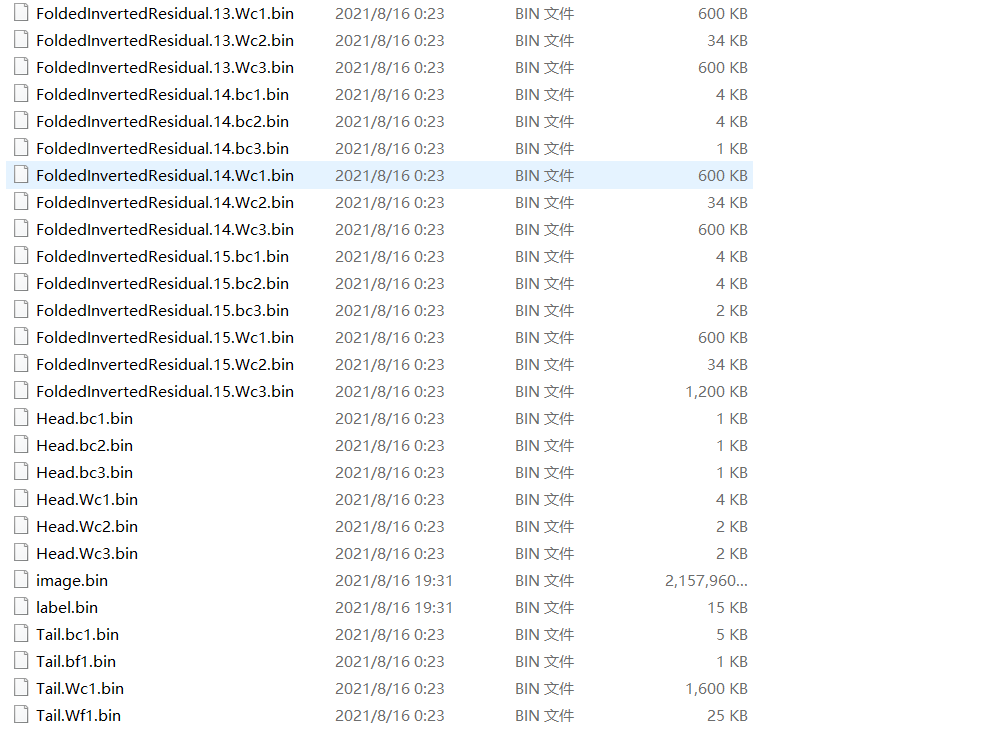# 加速器HLS设计

由于MobileNet V2由多个计算构成，因此我们设计了多个IP，分别加速不同的运算，主要有:
pwconv:加速point-wise卷积
dwconv:加速depth-wise卷积
conv:加速网络第一层的标准3x3s2卷积（网络第一层计算量也很大，因此也得加速，很无奈)
fc:加速全局平均池化层和全连接层
工程代码如下: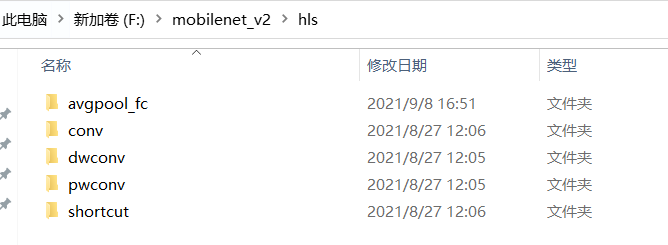这里，我们着重看dwconv和pwconv的设计。

## dwconv

dwconv，depth-wise卷积，一个通道对应一个卷积核，因此没有标准卷积中的通道求和操作，如下图所示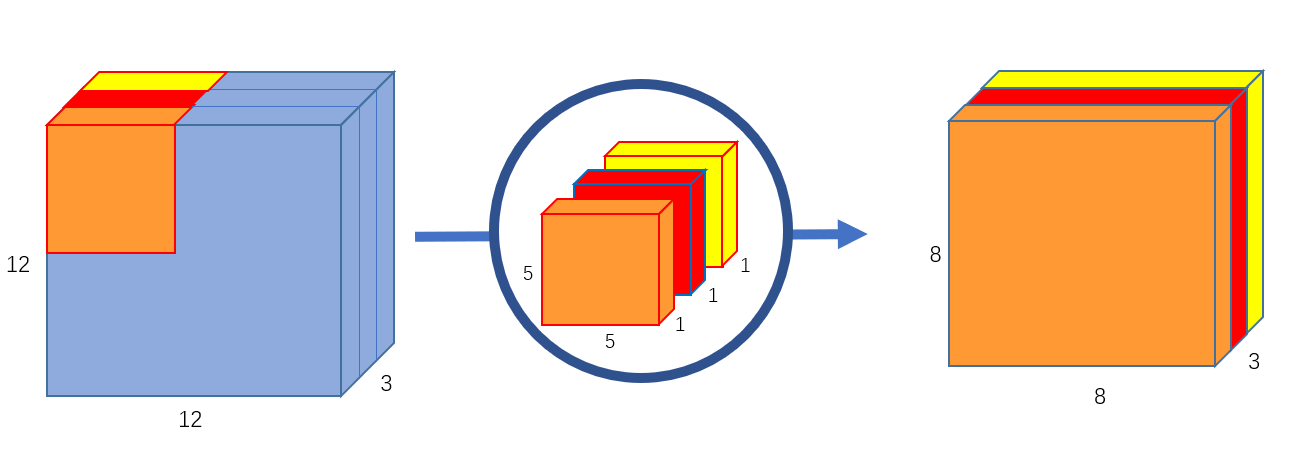我们将该卷积过程分为三个阶段:加载数据、计算卷积以及写回结果，为了掩盖数据的传输时间，我们进行了粗粒度流水线，即乒乓(双缓冲)操作，部分代码如下:

//
bool pp=true;
//阶段1
//阶段2
com_block.ch=0;
com_block.row=0;
com_block.col=0;
compute(fm_in_buff1,wt_buff1,bias_buff[com_block.ch/Tm],fm_out_buff1,stride);
//
update_block(o_fm_size,com_block);
store_block.ch=0;
store_block.row=0;
store_block.col=0;
while(1){
#pragma HLS LOOP_TRIPCOUNT min=62 max=62 avg=62
break;
if(pp){
compute(fm_in_buff2,wt_buff2,bias_buff[com_block.ch/Tm],fm_out_buff2,stride);
store_output(out1,out2,fm_out_buff1,fm_size,store_block,stride);
pp=false;
}
else{
compute(fm_in_buff1,wt_buff1,bias_buff[com_block.ch/Tm],fm_out_buff1,stride);
store_output(out1,out2,fm_out_buff2,fm_size,store_block,stride);
pp=true;
}
}
if(pp){
compute(fm_in_buff2,wt_buff2,bias_buff[com_block.ch/Tm],fm_out_buff2,stride);
store_output(out1,out2,fm_out_buff1,fm_size,store_block,stride);
//
store_output(out1,out2,fm_out_buff2,fm_size,store_block,stride);
}
else{
compute(fm_in_buff1,wt_buff1,bias_buff[com_block.ch/Tm],fm_out_buff1,stride);
store_output(out1,out2,fm_out_buff2,fm_size,store_block,stride);
//
store_output(out1,out2,fm_out_buff1,fm_size,store_block,stride);
}


这里我们并没有直接使用DATAFLOW指令，而是通过显示的if-else乒乓变量实现，综合结果表明，乒乓操作生效了，如下图所示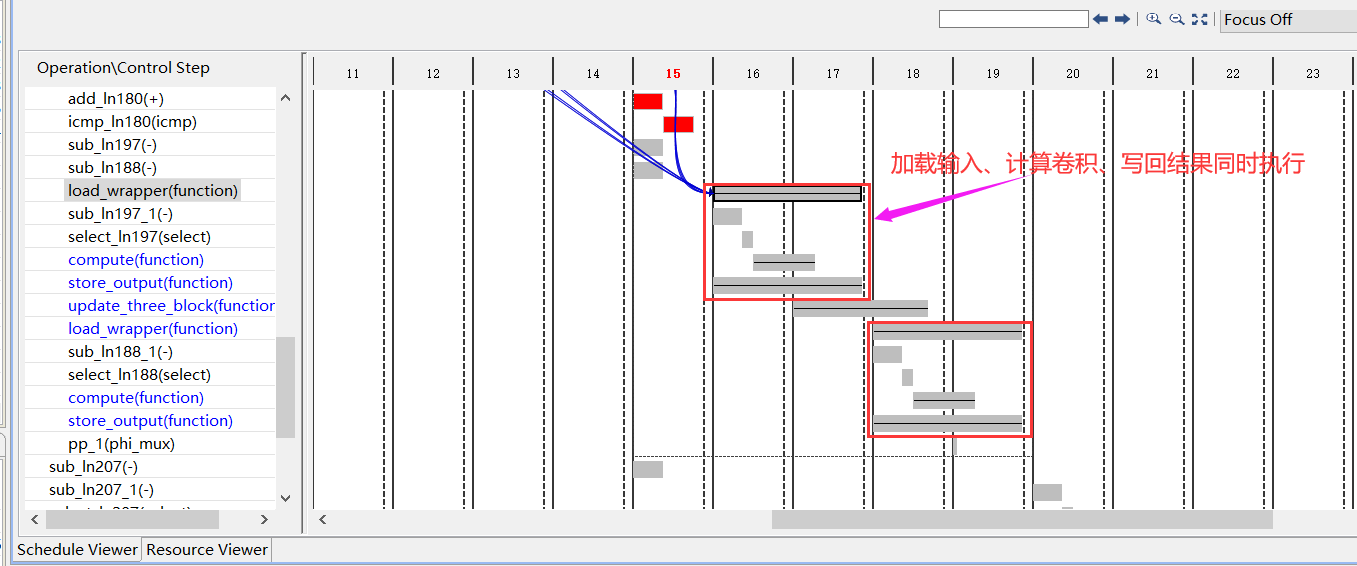同时，为了加快数据传输，我们将接口位宽增大为64bit，可一次性传输4个16bit的定点数，此外，我们还是用了多个传输接口，以进一步增大数据传输的带宽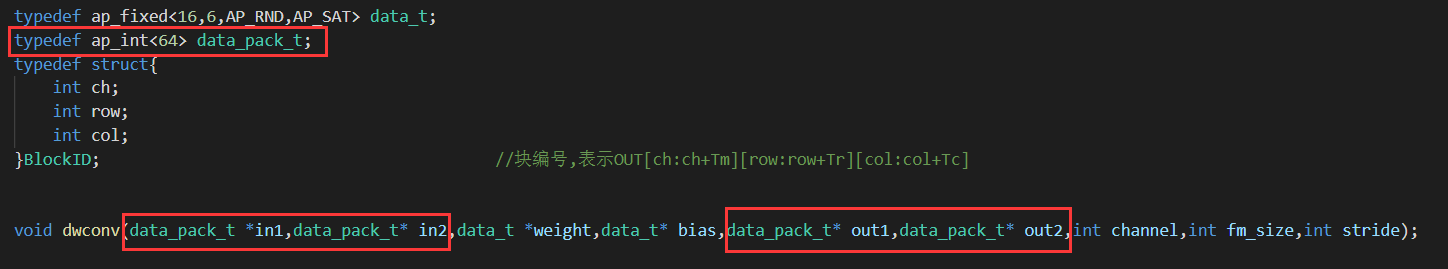## pwconv

pwconv,即1x1的标准卷积，它不改变特征图的大小，只改变特征图的通道数，在MobileNet V2中，它起着升维和降维的作用，此外，mobilenet v2的主要计算量都集中在pwconv，因此，加速pwconv是最重要的。
然而，不同于3x3标准卷积，pwconv实际上就是一个矩阵乘法，其计算/访存比较低，是memory-bounded的，因此，设计的思路主要就是增大数据传输的带宽，包括增大接口位宽增大突发传输长度以及增加接口数目等。
下面是加载输入特征的代码：

void load_input(data_t fm_in_buff[Tn][Tp],data_pack_t* in1,data_pack_t* in2,unsigned short n,unsigned short basePixAddr,unsigned short fm_size,bool flag){
unsigned short nn;
unsigned short i;
int length;
if(flag){
length=49;
}
else{
length=Tp;
}
for(i=0;i<length;i++){
#pragma HLS PIPELINE
for(int k=0;k<Tn/2;k++){
#pragma HLS UNROLL
fm_in_buff[k][i].range(15,0)=tmp1.range(16*k+15,16*k);
fm_in_buff[4+k][i].range(15,0)=tmp2.range(16*k+15,16*k);
}
}
}


可以看到，为了增加数据传输带宽，这里使用了两个接口(in1,in2)来并行的读取数据，并且每个接口，位宽都是64bit的，和HP接口的最大64bit相匹配，同时，两个接口的数据读取都是地址连续的，这样可以通过较长的突发传输长度，来抵消读地址通道握手所需的时间以及第一个数据读出所需等待的时间的开销。
和dwconv一样，pwconv也通过乒乓操作来掩盖数据的传输时间：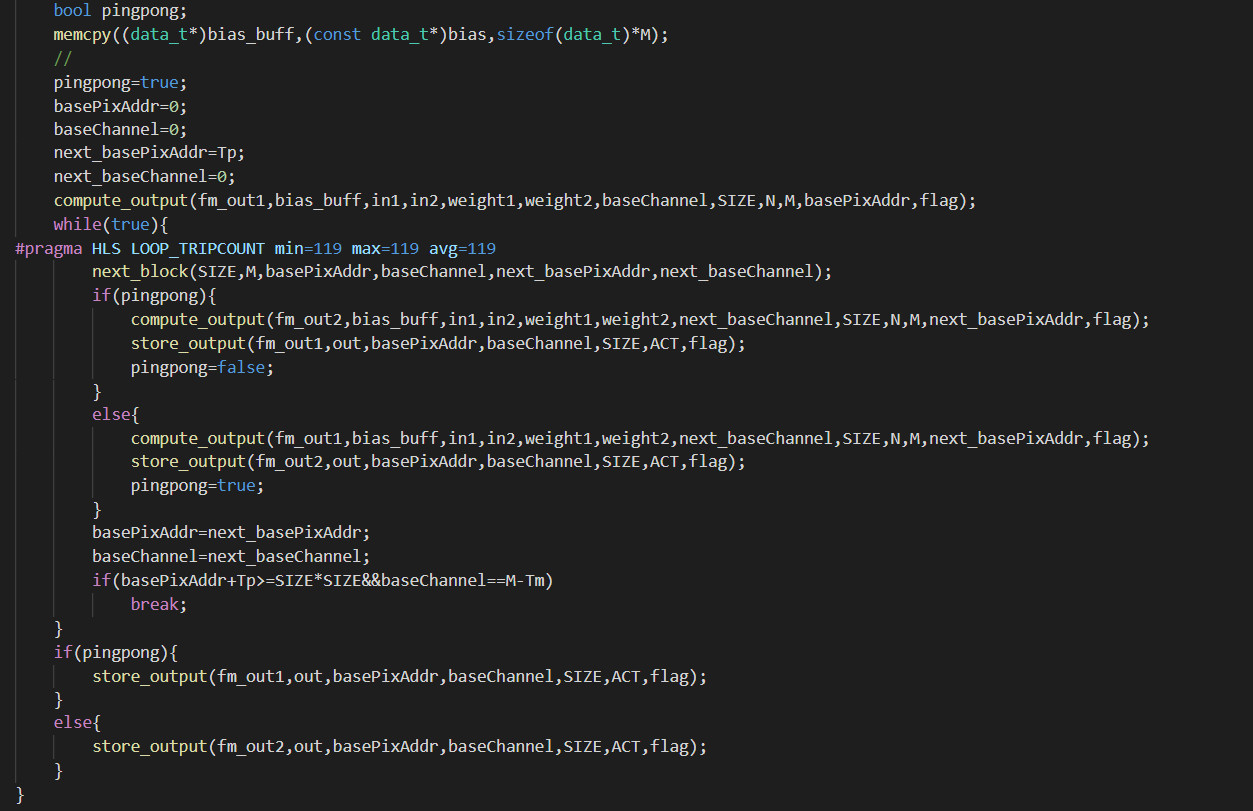# 硬件设计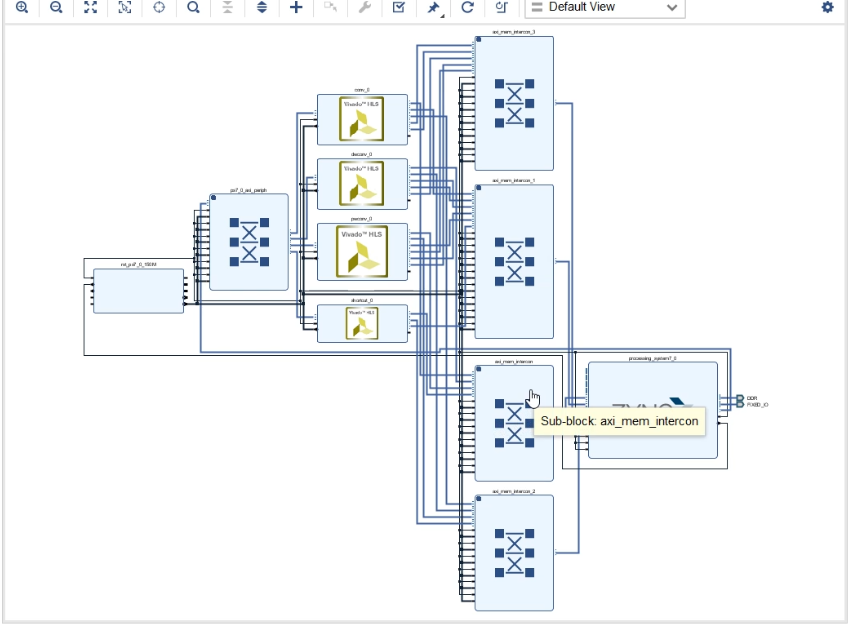综合、实现、生成bitstream

# SDK设计

SDK代码如下，这里就不一一列出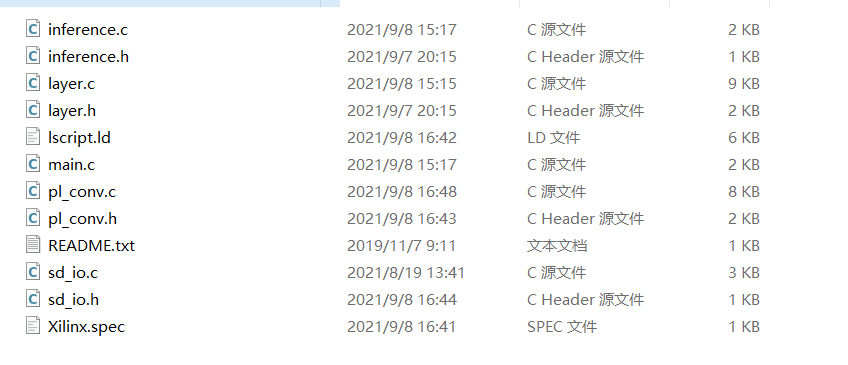数据集和权重被存储在SD卡上，我们通过xilinx提供的ff.h中的API进行数据的读取，以下是整个工程的main文件

#include"inference.h"
#include"pl_conv.h"
#include "xtime_l.h"
#pragma GCC optimize(3,"Ofast","inline")

int main()
{
SD_Init();          //import!
pl_pwconv_init(&hls_pwconv);
pl_dwconv_init(&hls_dwconv);
pl_shortcut_init(&hls_shortcut);
pl_conv_init(&hls_conv);
pl_fc_init(&hls_fc);
int N=250;
NetParam_q *netParam=(NetParam_q*)malloc(sizeof(NetParam_q));
SpaceAllocateq(netParam);
short* image=(short*)malloc(sizeof(short)*(N*3*224*224));
float* label=(float*)malloc(sizeof(float)*N);
int correct=0;
short *out=(short*)malloc(sizeof(short)*5);
Xil_DCacheFlush();
printf("N=%d\n",N);
XTime tEnd, tCur;
u32 tUsed;
for(int i=0;i<N;i++){
printf("i=%d\n",i);
XTime_GetTime(&tCur);
inference_q(image+i*3*224*224,netParam,out);
XTime_GetTime(&tEnd);
tUsed = ((tEnd-tCur)*1000000)/(COUNTS_PER_SECOND);
xil_printf("total time elapsed is %d us\r\n",tUsed);
float max_idx=-1;
int max_value=-9999;
for(int k=0;k<5;k++)
if(out[k]>max_value){
max_value=out[k];
max_idx=k;
}
if(max_idx==label[i])
correct++;
printf("%d,%d\n",(int)max_idx,(int)label[i]);
}
printf("test accuracy is %f\n",correct/(float)N);
SpaceDeleteq(netParam);
return 0;
}



注意:不要忘了修改堆栈大小，否则无法读取这么多测试数据，整个程序会卡住

# 结果

我们测试了250张图片，下图的结果表明，每张图片推理用时约为91ms，推理的准确率为98%(显然这个推理时间还能继续提升(只用了7020的一半资源))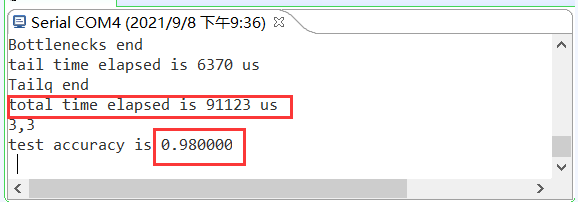展开全文• PS：只是模拟FPGA实现时的定点数量化训练过程… 哪个大佬有好的量化方案，求给个连接，官网的pytorch量化训练教程看不懂，以后看懂了再更吧 目录对pytorch的训练参数进行定点量化：1.建立模型2.查看模型参数3.转换...pytorch 机器学习
• 【超详细教程(附源码)】用FPGA加速卷积神经网络CNN运算 原创教程，转载请联系作者并注明出处：https://github.com/WalkerLau 源码地址：https://github.com/WalkerLau/Accelerating-CNN-with-FPGA 最近发现很多小...深度学习 人工智能芯片
• 基于Caffe中CIFAR_10.prototxt网络，对CIFAR-10数据集分类。 CIFAR_10.prototxt网络 name: "CIFAR_10" layer { name: "data" type: "Input" top: "data" input_param { shape: { dim: 1 dim: 3 dim: 32 dim: ...
• 基于FPGA的卷积神经网络硬件加速器设计空间探索研究.pdf
• 听完很多大咖的观点，对于工程师，未来十年应该有两个技术可以做，一个是AI，提升生产力，一个是区块链，厘清生产关系。个人觉得还是AI好玩些，希望可以挤进去折腾下。对于AI应用落地，其实判断...FPGA工程师如果想...
• 基于改进动态配置的FPGA卷积神经网络加速器的优化方法.pdf
• 针对卷积神经网络(CNN)在通用CPU以及GPU平台上推断速度慢、功耗大的问题，采用FPGA平台设计了并行化的卷积神经网络推断系统。通过运算资源重用、并行处理数据和流水线设计，并利用全连接层的稀疏性设计稀疏矩阵乘法...
• ABM-SpConv：一种基于FPGA的卷积神经网络推理加速方法研究论文
• 现有的卷积神经网络由于其结构复杂且依赖的数据集庞大，难以满足某些实际应用或者计算平台对运算性能的要求和能耗的限制。...该实现方式在GOPS、能源和资源效率方面均优于现有的FPGA神经网络加速方法。
• 这是浙大余子健的研究生毕业论文，也是我阅读的第一篇文献。...卷积层是FPGA加速的重点。 上图是一个典型的卷积层，N个输入特征图，M个输出特征图，将会有N*M个卷积核 (Kernals)。这点我觉得很重要：...
• 特征图并行：由细粒度到粗粒度 （1）卷积窗口内部，3*3卷积 （2）同一特征图上，卷积窗口的滑动 （3）不同卷积窗口对应的不同特征图 （4）不同个数的卷积通道对应输入的特征图 （1）窗口内部卷积实现 ...
• 本文将阐释深度学习和FPGA各自的结构特点以及为什么用FPGA加速深度学习是有效的，并且将介绍一种递归神经网络（RNN）在FPGA平台上的实现方案。　揭开深度学习的面纱　深度学习是机器学习的一个领域，都属于人工智能...
• 该文档为基于FPGA总线框架的深度卷积神经网络加速器关键技术研究讲解文档，是一份很不错的参考资料，具有较高参考价值，感兴趣的可以下载看看………………
• 基于FPGA的卷积神经网络加速器设计与实现 (1).pdf
• 递归神经网络(RNN)近些年来被越来越多地应用在机器学习领域，尤其是在处理序列学习任务中，相比CNN等神经网络性能更为优异。但是RNN及其变体，如LSTM、GRU等全连接网络的计算及存储复杂性较高，导致其推理计算慢，很...
• 基于FPGA的卷积神经网络加速方案设计 ，钟楠，刘明，卷积神经网络（CNN）已广泛应用于计算机视觉，然而计算机有限的计算能力对大规模的CNN的实现带来许多挑战。针对CNN并行性计算的特点
• 发明名称：一种基于FPGA的卷积神经网络硬件加速架构 摘要 本发明公开一种基于FPGA的卷积神经网络硬件加速架构，包括：通用AXI4总线接口；用于缓存输入特征图，输出特征图和权重的缓存区；用于引导运算结果缓存的...
• 以下主要引用自西安邮电大学李涛老师关于连接智能和符号智能的报告，以及fpl2016上ASU的 Yufei Ma的...Scalable and Modularized RTL Compilation of Convolutional Neural Network onto FPGA 地址：http://fpl2016....
• 针对该问题，根据卷积神经网络计算模式的特点，提出一种面向云端FPGA的卷积神经网络加速器的设计及其调度机制。通过借鉴基于HLS技术、引入循环切割参数和对卷积层循环重排的设计，采用模块化方式构造网络，并进行......